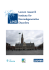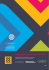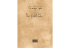# Tilburg University Controlled communication networks Feltkamp, V

## Transcription

Tilburg University Controlled communication networks Feltkamp, V
```Tilburg University
Controlled communication networks
Feltkamp, V.; van den Nouweland, C.G.A.M.
Publication date:
1992
Citation for published version (APA):
Feltkamp, V., & van den Nouweland, C. G. A. M. (1992). Controlled communication networks. (Research
memorandum / Tilburg University, Department of Economics; Vol. FEW 538). Unknown Publisher.
General rights
Copyright and moral rights for the publications made accessible in the public portal are retained by the authors and/or other copyright owners
and it is a condition of accessing publications that users recognise and abide by the legal requirements associated with these rights.
? Users may download and print one copy of any publication from the public portal for the purpose of private study or research
? You may not further distribute the material or use it for any profit-making activity or commercial gain
? You may freely distribute the URL identifying the publication in the public portal
Take down policy
~ ~M ~r~~
~~
7626 ~ ~~, ~,~~~ ~
1992 ~p ~~, ~~
538
,
~
~
~~~ J~U ~~~
~p ~`~~ ~~~
I III~I I IIII IIIII INf~IIINNIn~ll~ll ll
o~~~ooo~~.`~`~~~~
e
~j ~
,
,
,~
,
.,
,í
.
C
N
I
,
0
1
9
CONTROLLED CONIN1tJNICATION NETWORKS
V. Feltkamp
A. van den Nouweland
~ 538
Refereed by Prof.dr. S.H. Tijs
,~
~.
r~.~~ ~~.~~
G
Controlled Communication Networks
By V. Feltkamp` and A. van den Nouweland'
Abstract
This paper considers a generalisation of communication situations, namely controlled communication networks. Three solution concepts for controlled communicatíon networks are introduced, the Myerson value, the position value and the
mixed value, which are inspired by corresponding solution concepts for communication situations, and a~ciomatic characterisations of these concepts are provided.
Further, network games associated with controlled communication networks are
considered and it is shown that every TU-game can be obtained as a network
game.
' Depsrtment of Econometrics, Tilburg University, P.O. Box 90153, 5000 LE Tilburg, The Netherlaada
2
1
Introduction and Preliminaries
In a cooperative TU (Transferable Utility) game (N, v) as modelled by von Neumann
8t Morgenstern (1944), N is a finite set of players, and the chazacteristic function v
assigns to each subgroup of players a real number which is to be interpreted as the
maximal gains (minimal costs) this coalition can achieve by cooperating, regardless of
the actions of the other players.
It is generally assumed that each subgroup of players can form and cooperate to obtain
its value. However, this approach fails to take into account communication restrictions
that may cause deficiencies in cooperation in some coalitions. Myerson (1977) introduced
communication graphs to model non-transitive communication restrictions. In such a
graph the vertices are the players and an edge between two players represents the fact that
these players can communicate directly. The general procedure is the following: given
a TU-game (N, v) and a communication graph (N, A), one defines a rewazd function
r on collections of vertices and edges which takes the communication restrictions into
account, and then new games are extracted. This model was elaborated further by Owen
(1986), Borm, Owen 8a Tijs (1992), Van den Nouweland, Borm, Owen and Tijs (1991)
and Van den Nouweland 8z Borm (1991). A survey on this subject is given in Borm, Van
den Nouweland 8s Tijs (1991).
In this paper, we generalise communication situations by allowing the vertex set of
the graph to differ from the set of players N, and by starting out with a reward function
r, instead of deriving it. This means that we can assign different rewards to different
connected graphs with the same vertex set. We assume that the players control the
vertices and edges of the graph through so-called control games. In section 2 we provide
the formal definition of controlled communication networks and we introduce three solution concepts for these networks, the Myerson value, the position value and the mixed
value. These solution concepts are characterised axiomatically in section 3. Finally, in
section 4 we present an alternative way of constructing a TU-game correeponding to a
controlled communication network, and we show that all TU-games can be obtained as
such a network game.
Preliminaries
Let N:- {1,...,n}, and 2N :- {S ~ S C N}. We denote the class of all transferable
utility games with player set N by TUN.
For each S E 2N`{~}, the unanimity game (N, us) E TUN is defined by
3
us(T) -
~ 1
0
ifSCT
otherwise
for all T C N.
It is well known that {(N, us) ~ S E 2N`{~}} forms a basis of TUN.
The Shapley value ~(cf. Shapley, 1953) is a linear function TUN -. RN defined by
~;(N~ us) - ~ ~S~
0
if
iES
if i E N`S
for all S E 2N`{0}.
2
Controlled Communication Networks
Consider a finite undirected graph ( V, E) without loops or parallel edges. We assume
that for each vertex v E V a simple control game (N, c„) with veto players is given and,
similarly, for each edge e E E a simple control game (N, c~) with veto players is given.
Here, a TU-game (N,c) is called simple if c(S) E {0,1} for all S C N and c(N) - 1.
Moreover, a player i E N is called a veto player of the simple game (N, c), denoted by
i E veto(c), if i can block a positive outcome, i.e. c(S) - 0 for all S E 2N`{~} with
i~ S. If c„ is the control game for vertex v E V, then a coalition S C N is allowed to
use vertex v if and only if c„(S) - 1. The control games (N, c~) for edges have a similar
interpretation.
Furthermore, we assume that there is a reward function r on subsets of vertices and
edges r: 2~ x 2E --~ R, measuring the economic value of subnetworks. Keeping in
mind that edges are to model communication channels, it seems reasonable to assume
that an edge is useless without both its end points, i.e. for all W C V and F C E
it holds that r(W,F) - r(W,F`{{vl,vz}}) if {vl,v~} E F is such that {vl,vz} is not
a subset of W.
So, the reward of a network (W, F) does not depend on the edges
not in F(W) :- {{v,w} E F ~ v E W,w E W}.
Moreover, in a network (W,F),
W is partitioned into communication components in the following way: C C W is a
component within (W, F) if and only if (C, F(C)) is a connected subgraph of (W, F(W))
and is maximal with respect to this property. The resulting partition of W is denoted
W~ F.
Correspondingly, we assume that the reward function is additive with respect to these
components, i.e.
4
r(W, F) -
~ r(C, F(C))
CEw~F
for all W C V and all F C E.
For simplicity, we assume that r is zero-normalised, i.e. r({v},0) - 0 for all v E V.
A controlled communication network is a 6-tuple (N, V, E, {c„~v E V}, {c~~e E E}, r)
as described above. The set of all controlled communication networks with player set N
will be denoted CCNN.
The purpose of this paper is to present and characterize some solutions to the problem
of how to distribute the reward r(V, E) among the players in N. Formally, a solution
concept on CCNN is a function ry : CCNN -~ RN assigning y;(C) to player á in the
controlled communica.tion network C E CCNN. One way to obtain solution concepts
on CCNI" is to construct for each controlled communication network a TU-game corresponding to this network in which the players are the edges and~or Vertices of the graph.
To this game one can apply a solution concept from cooperative game theory, for example the Shapley value. This yields a Value for edges and~or vertices and this Value can
be distributed among the players according to veto control. Concentrating on vertices
this procedure yields the Myerson value (cf. Myerson, 1977) and concentrating on edges
it yields the position value (cf. Borm, Owen, and Tijs, 1992). If no such distinction
between vertices and edges is made, one obtains the mixed Value.
Let C- (N,V, E, {c„~v E V}, {c~~e E E},r) be a controlled communication network.
Then the Myerson value p(C) E RN is defined by
f~~(C) :-
~
vEV:iEveto(c~)
~v(y, rE)
~ veto(c„)~
for all i E N, where the vertex game (V, rE) is a game in which the Vertices are the
players, defined by rE(W) - r(W,E) for all W C V.
Further, the position value z(C) E RN is defined by
a;(C) :-
~
~`(E'rv)
eEE:iEveto(c.) I VetO(Cc)I
5
for all i E N, where the edge game ( E, ry) is a game in which the edges are the players,
defined by ry(F) - r(V, F) for all F C E.
Finally, the mixed yalue p(C) E RN is defined by
p~(C) .-
~
j E vUE:i Eveto(c~ )
~j(V U E, r)
~ veto(cj)~
for all i E N, where the game (V U E, r) is defined by r(J) - r(J fl V, J fl E) for all
JCVUE.
3
Axiomatic characterisations
In this section we provide axiomatic characterisations of the three solution concepts introduced in section 2. It will turn out that all three concepts can be characterised by
four axioms, three of them being the same for all three solution concepts.
For concision, if C is a controlled communication network and F C E a set of edges we
will use C-F to denote the network where the edges in F have been omitted, and where
the reward function has been restricted accordingly. We now introduce some properties.
A solution concept ry on CCNN is called efGcient if for each controlled communication
network C, ry distributes exactly r(V, E) among the players. In formula:
~ ry;(C) - r(V, E).
~EN
A solution concept ry on CCNN is called additive if it is additive with respect to the
reward function ( ceteris paribus).
A solution concept ry on CCNN is said to have the superfluous edge property if for all
C E CCNN and all edges e E E that are superfluous for C it holds that
7(C) - 7(C-{~}).
Here, an edge e E E is called superfluous for C if for all F C E,
r(V, F) - r(V, F`{e}).
6
Note that for an edge e to be superfluous, we only consider the whole set of vertices V;
we do not demand r(W, F) - r(W, F`{e}) for all W C V and F C E. However, this
turns out to be an equivalent demand.
Lemma 1 Let C E CCNN. Then an edge e E E is superfluous for C if and only if
r(W, F) - r(W, F`{e})
forallWCVandaIlFCE.
Proof. The "if~ part is straightforward. For the ronly if" part, note that
r(W, F)
-
~
r(C, F(C))
CEW~F
-
~
r(C, F(C))
CE w~F(W )
~
(')
r(C, F(C)) - r(V, F(W))
CEV~F(W)
for all F C E and W C V.
Here, equality (~) follows from the fact that r is zero-normalised.
Hence, for a superfluous edge e
r(W, F`{e})
-
r(V, (F`{e})(W))
-
r(V, F(W)`{e})
-
r(V, F(W))
- r(W, F)
for all F C E and W C V. This competes the proof.
O
In a graph (V, E), we denote by D(V, E) the set of vertices that have at least one neighbo~ir in the graph, and we will shorten this notation to D whenever this does not lead
to confusion.
7
The fourth property of solution concepts on CCNN we introduce is anonymity. A
controlled communication network is said to be anonymous if the reward function only
depends on the number of non-isolated vertices and edges, i.e. there exists a function
f:{0,..., ~D U E~} --~ R such that
r(W, F) - f(~(W fl D) U F~)
for all W C V and F C E.
A solution concept ry on CCNN satisfies anonymity if for all anonymous C E CCNN,
the solution is proportional to the veto power of the players over the non-isolated parts
of the graph or, in formula: there exists an a E R such that for all i E N
ry(C)-a'
`
1
~
jEDUE:iEreto(ej) I VetO(Cj)I
The mixed Value p satisfies the four properties mentioned. This is shown in
Lemma 2 Tl~e mixed value p satisfies efficiency, additivity, anonymity and the superfluous edge property.
Proof. Let C be a controlled communication network. Then
~ Pi(C)
-
iEN
~
~j(V U E,r)
~
I VetO(Cj)I
iEN jEVUE:iEveto(ej)
~ VUET
-
-
jE~E
'(
~
~
)
1
iEveto(e~) I VetO(Cj)I
~~j(V U E, r`) (-) r(V, E),
j EVUE
where equality (~) follows from efficiency of the Shapley value ~. Hence, p is ef6cient.
In order to prove the superfluous edge property, take C E CCNN and e E E that is
superfluous for C. It clearly suffices to prove that ~~(V U E, r') - 0 and ~j (V U E, r") ~j(VUE`{e}, r) for all j E VUE`{e}. Using Lemma 1 we easily obtain r"(J) - r"(J`{e})
for any J C V U E. Hence, e is a zero playet in the game (V U E, r`), and consequently
~~(V U E,r`) - 0 and ~j(V U E,r`) -~j(V U E`{e},r) for all j E V U E`{e}.
We
8
conclude that p satisfies the superfluous edge property.
Now let C E CCNN be anonymous, and let f:{0,..., ~D U E~} -~ R be such that
r(W, F) - f(~(D fl W) U F~) for all F C F, and W C V. Then all vertices v E V`D are
zero players in the game (V U E, r), and all j E D U E are symmetric in this game. By
symmetry, efiiciency and the dummy property of ~ this implies
~j(V U E,T)
Hence, Pi(C) - a'
-{
~E
~DuE~
0
if'EDUE
~
ifjEV`D.
~
~~~t~~,)I'
1EDUE:iE~eto(e~)
where a:-
~ouÉ~
.
O
Before we prove that p is characterised by the four properties,we introduce two more
definitions.
Let (V, F.) be a graph. Then we denote by ?Z(V, E) the set of (V, F.)-admissible reward
functions, i.c.
R(V, E) :- {r : 2~ x 2E -~ R ~ r is additive w.r.t. components and zero-normalised}.
Moreover,
B(V, E)
:-
{uiy,F : 2V x 2E -~ R ~ (W, F) is a connected subgraph of(V, E),
and ~W~ ~ 2},
where uyy,F is defined by
uiy,F(W', F') :
-~ 1
0
ifWCW'andFCF'
otherwise.
Then we have the following
Lemma 3 Let (V, E) be a fixed graph. Then li(V, E) forms a basis of the vector space
1Z(V, E).
This lemma is an easy corollary of the fact that the set of unanimity games
{(N,us) ~ S E 2N`{0}} forms a basis of TUN, and that the reward functions are zeronormalized. However, the proof is rather technical, and therefore we omit it.
9
Theorem 4 The mixed value p is the unique solution concept on CCNN satisfying
effïciency, additivity, anonymity and the superfluous edge property.
Proof. According to Lemma 2 p satisfies the four properties. Hence, we only have to
show that there is at most one solution concept satisfying these properties. Suppose ry
is a solution concept on CCNN that satisfies the four properties. Using lemma 3 and
additivity of ry and p, we see that it suffices to prove ry - p for situations in which
r- Qux;F for some Q E R and some connected subgraph (W, F) with ~ W ~~ 2. Hence,
let C C CCNN be a controlled communication network with r- Qury,F for some ~i E R
and some connected subgraph (W, F) with ~ W ~~ 2.
Since every edge e in E`F is
superfluous for C, the superfluous edge property implies that ry(C) - ry(C-E`F).
Furthermore,
r(W~, F~) - Quw,F(W~~ F~) -
Q ifWCW'andF'-F
0
otherwise
for all W' C V and F' C F.
Since (W, F) is a connected graph, it holds that D(V, F) - W. So, defining
J: {0,...,~DUE~}--~Rby
J(k) -{,B
0
if k- ~W U F~
otherwise
we see that the controlled communication network C-E~F is anonymous.
Now, by
anonymity of ry we know that there exists an a E R such that
1
i
ry~(C-E`F)
- ~ '
L
jEWUF:iEveto(cj) I VetO(Cj)I
for all i E N.
Using efficiency, we obtain
[~
,B - r(V, F) -~ ry~(C-E`F) - L. ~'
iEN
iEN
1
-~' I W U F~.
jEWuF:iEveto(e~) I veto ( cj)I ~
Hence, ~- Q - ~W U F~-' and recalling ry(C) - ry(C-~F), we see
10
7i(C) - IW
U F~
~
7 EW uF:i Eveto(c~ )
Pi(C).
~ veto(ci)~ -
We proceed by providing axiomatic characterisations of both the Myerson value and the
position value. Both values can be characterised by efficiency, additivity, the superfluous edge property and an anonymity axiom. The anonymity axioms we need are vertex
anonymity and edge anonymity.
A solution concept ry on CCNN
is said to be vertex anonymous if for every
controlled communication network C E CCNN such that there exists a function
f:{0,..., ~D(V,E)~} -~ R with r(W,E) - f(~D fl W~) for all W C V, there is an
~ E R such that for all i E N
ry;(C) - ~ .
~
~ veto(c„)~-1.
vED:iEveto(e~ )
A solution concept ry on CCNN is called edge anonymous if for every C E CCNj" such
that there exists a function f: {0,..., ~E~} --~ R with r(V,F) - f(~F~) for all F C E,
there is an a E R such that for all i in N
7i(C) - ~ -
~
~ veto(c~)~-1.
eEE:iEveto(c~)
Theorem 5
i) The Myerson value p is the unique solution concept on CCNN that satisfies ef~iciency, additivity, the superfluous edge property and vertex anonymity.
ii) The position value ~r is the unique solution concept on CCNN that satisfies efficiency, additivity, the superfluous edge property and edge anonymity.
The proof of theorem 5 runs along the same lines as the proof of lemma 2 and theorem
4 and therefore it is left to the reader.
Remark. The axiomatic characterisations of the Myerson value and the position value
provided in theorem 5 are similar to axiomatic characterisations provided in Borm, Owen
11
and Tijs (1992) for TU-communication situations. However, the reader should note that
our characterisations hold for all controlled communication networks whereas Borm,
Owen and Tijs (1992) had to restrict to cycle-free communication graphs.
4
Network games
In the previous sections we approached the problem how to divide the reward r(V, E)
of a controlled communication network amongst the players in an indirect way, by first
determining the value of vertices and edges and then distributing these values among
the veto players in the corresponding control games. In this section we will describe a
direct way of dealing with the problem.
Let C be a controlled communication network. Now we define an associated game
with player set N in the following way:
Let S C N. Then V(S) :- {v E V ~ c„(S) - 1} is the set of all vertices that coalition
S can control, and E(S) :- {e E E ~ c~(S) - 1} is the set of edges that coalition S can
control. Correspondingly, coalition S can obtain
vc(S) :- r(V(S),E(S)).
Hence, we associate with C E CCNN the network game (N, vc) as defined above.
Consequently, some solution concept for TU-games could be applied to the game (N, vc).
This approach seems interesting, because a number of games associated with economic
situations can be seen to be network games in a more or less natural way. Some examples
are sequencing games (Curiel, Pederzoli 8z Tijs, 1989), permutation games (Tijs et al.,
1984) and assignment games (Shapley 8s Shubik, 1972).
However, some scepticism is in place here, because every TU-game is a network game in
a trivial way:
Let (N, v) be an arbitrary TU-game. We proceed by defining a controlled communication network corresponding to (N,v). Let V:- N and E:- {{i, j} ~ i, j E N,i ~ j}.
Hence, (V, E) is the complete graph with vertex set N. The control game for each vertex
i E N is (N,u{;}) and the control game for each edge {i, j} is (N,u{;,~}).
Hence, every
player is a dictator for his own vertex and an edge between two vertices is controlled by
12
Lhc two playc,rs iL conn~c.Ls. Finally, thc reward function r a.vsigns n(.S) t.o thc aubgraph
(S, E(S)) for all S C N, and is extended in some feasible way to all subsets of vertices
and edges. It is easily seen that the network game associated with the controlled communication network described above is the game (N, v).
Note that in the above discussion we did not restrict to zero-normalised games. However, the restrictions to zero-normalised reward functions was only made for simplicity
and is not essential. Hence, we do not have to worry about it here.
13
References
Borm, P., Owen, G., and Tijs, S.H. (1992). ~On the position value for communication
situations", to appear in SIAM J. Discr. Math.
Borm, P., Nouweland, A. van den, and Tijs, S.H. (1991). "Cooperation and communication restrictions: a surveyn, in: 'Economic Behaviour in an Imperfect Environment', Gilles, R.P. and Ruys, P.H.M. (Eds.), North-Holland, Amsterdam. (To
appear)
Curiel, I., Pederzoli, G., and Tijs, S.H. (1989). „Sequencing games", Eur. J. Oper.
Res. 40, 344-351
Myerson, R.B. (1977).
"Graphs and cooperation in gamesn, Math.
Oper.
Res. 2,
225-229.
Neumann, J. von and Morgenstern, O. (1944).
nTheory of Games and Economic
Behavior„ (3rd edition, 1953), Princeton University Press, Princeton.
Nouweland, A. van den and Borm, P. (1991). "On the convexity of communication
gamesM, Int. J. Game Th. 19, 421-430.
Nouweland, A. van den, Borm, P., Owen, G., and Tijs, S.H. (1991). "Cost allocation
and communicationn, Res. Mem. FEW 469, Dept. of Econometrics, Tilburg
University, The Netherlands.
Owen, G. (1986). „Values of graph-restricted games", SIAM J. Alg. Discr. Meth. 7,
210-220.
Shapley, L.S. (1953). „A value for n-person games", in: Contributions to the Theory of
Games, II (Eds. Tucker, A.W. and Kuhn, H.), Ann. Math. Studies 28, Princeton
University Press, 307-317.
Shapley, L.S. and Shubik, M. (1972). rThe assignment game I: the core", Int. J. Game
Th. 1, 111-130.
Tijs, S.H., Parthasarathy, T., Potters, J., and Rajendra Prassad, V. (1984). "Permutation games: another class of totally balanced games„, OR Spectrum 6, 119-123.
i
IN 19)1 REEDS VERSCHENEN
466
Prof.Dr. Th.C.M.J. van de Klundert - Prof.Dr. A.B.T.M. van Schaik
Economische groei in Nederland in een internationaal perspectief
467
Dr. Sylvester C.W. Eijffinger
The convergence of monetary policy - Germany and France as an example
468
E. Nijssen
Strategisch gedrag, planning
binnen de computerbranche
en
prestatie.
Een
inductieve
studie
469
Anne van den Nouweland, Peter Borm, Guillermo Owen and Stef Tijs
Cost allocation and communication
470
Drs. J. Grazell en Drs. C.H. Veld
Motieven voor de uitgifte van converteerbare obligatieleningen en
471
P.C. van Batenburg, J. Kriens, W.M. Lammerts van Bueren and
R.H. Veenstra
Audit Assurance. Model and Rayesian Discovery Sampling
472
Marcel Kerkhofs
Identification and Estimation of Household Production Models
473
Robert P. Gilles, Guillermo Owen, René van den Brink
Games with Permission Structures: The Conjunctive Approach
474
Jack P.C. Kleijnen
475
Sensitivity Analysis
of Simulation Experiments:
sion Analysis and Statistical Design
Tutorial on Regres-
C.P.M. van Hoesel
An 0(nlogn) algorithm for the
controllable machine speeds
shop
two-machine
flow
problem
476
Stephan G. Vanneste
A Markov Model for Opportunity Maintenance
477
F.A. van der Duyn Schouten, M.J.G. van Eijs, R.M.J. Heuts
Coordinated replenishment systems with discount opportunities
478
A. van den Nouweland, J. Potters, S. Tijs and J. Zarzuelo
Cores and related solution concepts for multi-choice games
479
Drs. C.H. Veld
Warrant pricing: a review of theoretical and empirical research
480
E. Nijssen
De Miles
and
branche
481
Snow-typologie:
with
Een exploratieve studie in de meubel-
Harry G. Barkema
Are managers indeed motivated by their bonuses?
ii
482
483
484
Jacob C. Engwerda, André C.M. Ran, Arie L. Rijkeboer
Necessary and sufficient conditions for the existgnce of
definite solution of the matrix equation X. ATX- A- I
a
positive
Peter M. Kort
A dynamic
costs
model
of the firm with uncertain earnings and adjustment
Raymond H.J.M. Gradus, Peter M. Kort
Optimal taxation on profit and pollution
framework
within
a
macroeconomic
485
René van den Brink, Robert P. Gilles
Axiomatizations of the Conjunctive Permission Value for Games with
Permission Structures
486
A.E. Brouwer ~, W.H. Haemers
The Gewirtz graph - an exercise in the theory of graph spectra
487
Intratemporal uncertainty in the multi-good
model: motivation and application
life
cycle
consumption
488
J.H.J. Roemen
The long term elasticity of the milk supply with respect to the milk
price in the Netherlands in the period 1969-1984
489
Herbert Hamers
The Shapley-Entrance Game
490
Rezaul Kabir and Theo Vermaelen
Insider trading restrictions and the stock market
491
Piet A. Verheyen
The economic explanation of the jump of the co-state variable
492
Drs. F.L.J.W. Manders en Dr. J.A.C. de Haan
De organisatorische aspecten bij systeemontwikkeling
een beschouwing op besturing en verandering
493
Paul C. van Batenburg and J. Kriens
Applications of statistical methods and techniques to auditing and
accounting
494
Ruud T. Frambach
The diffusion of innovations: the influence of supply-side factors
495
J.H.J. Roemen
A decision rule for the ( des)investments in the dairy cow stock
496
Hans Kremers and Dolf Talman
An SLSPP-algorithm to compute an
linear production technologies
equilibrium
in
an
economy
with
iii
49~
L.W.G. Strijbosch and R.M.J. Heuts
Investigating several alternatives for estimating the compound lead
time demand in an (s,Q) inventory model
498
Bert Bettonvil and Jack P.C. Kleijnen
Identifying the important factors in
factors
499
Drs.
H.C.A.
Roest,
Drs.
F.L.
simulation
models
with
many
Tijssen
Beheersing van het kwaliteitsperceptieproces bij diensten door middel
van keurmerken
500
501
B.B. van der Genugten
Density of the F-statistic
normal distributed errors
in
the
linear
model
with
arbitrarily
Harry Barkema and Sytse Douma
The direction, mode and location of corporate expansions
502
Gert Nieuwenhuis
Bridging
the
distribution
gap
between
a
stationary point process and its Palm
503
Chris Veld
Motives for the use of equity-warrants by Dutch companies
504
Pieter K.
Jagersma
Een etiologie van horizontale internationale ondernemingsexpansie
505
B. Kaper
On M-functions and their application to input-output models
506
A.B.T.M. van Schaik
Produktiviteit en Arbeidsparticipatie
50~
Peter Borm, Anne van den Nouweland and Stef Tijs
Cooperation and communication restrictions: a survey
508
Willy Spanjers, Robert P. Gilles, Pieter H.M. Ruys
509
Martijn P. Tummers
The Effect of Systemstic Misperception of Income on the Subjective
Poverty Line
510
A.G. de Kok
Basics of Inventory Management: Part 1
Renewal theoretic background
511
J.P.C. Blanc, F.A. van der Duyn Schouten, B. Pourbabai
Optimizing flow rates in a queueing network with side constraints
512
R. Peeters
On Coloring j-Unit Sphere Graphs
iV
513
Drs. J. Dagevos, Drs. L. Oerlemans, Dr. F. Boekema
Regional economic policy,
economic
technological
networks
innovation
and
514
Erwin van der Krabben
Het functioneren van stedelijke onroerend-goed-markten in Nederland een theoretisch kader
515
Drs. E. Schaling
European central bank independence and inflation persistence
516
Peter M. Kort
Optimal abatement policies within a stochastic dynamic model
firm
517
518
Expenditure
tion model
of
the
versus consumption in the multi-good life cycle consump-
Large, infrequent consumption in the multi-good life
tion model
cycle
consump-
519
Pollution and Endogenous Growth
520
Arbeidsongeschiktheid, subjectief ziektegevoel en collectief belang
521
A.G. de Kok
Basics of inventory management: Part 2
The (R,S)-model
522
A.G. de Kok
Basics of inventory management: Part 3
The (b,Q)-model
523
A.G. de Kok
Basics of inventory management:
The (s,S)-model
524
Part 4
A.G. de Kok
Basics of inventory management:
The (R,b,Q)-model
Part 5
525
A.G. de Kok
Basics of inventory management: Part 6
The (R,s,S)-model
526
Rob de Groof and Martin van Tuijl
Financial integration and fiscal policy in interdependent
economies with real and nominal wage rigidity
two-sector
V
52~
A.G.M. van Eijs, M.J.G. van Eijs, R.M.J. Heuts
GecotSrdineerde bestelsystemen
528
M.J.G. van Eijs
Multi-item inventory systems with joint ordering
decisions
and
transportation
529
Stephan G. Vanneste
Maintenance optimization of a production system with buffercapacity
530
Michel R.R. van Bremen, Jeroen C.G. Zijlstra
Het stochastische variantie optiewaarderingsmodel
531
Willy Spanjers
Arbitrage
and Walrasian Equilibrium
mation
in Economies with Limíted
Infor-
Vi
IN 1992 REEDS VERSCHENEN
532
F.G. van den Heuvel en M.R.M. Turlings
Privatisering van arbeidsongeschiktheidsregelingen
Refereed by Prof.Dr. H. Verbon
533
J.C. Engwerda, L.C. van Willigenburg
LQ-control of sampled continuous-time systems
Refereed by Prof.dr. J.M. Schumacher
534
J.C. Engwerda, A.C.M. Ran ~ A.L. Rijkeboer
Necessary and sufficient conditions for the existence of
definite solution of the matrix equation X t ANX-lA - Q.
Refereed by Prof.dr. J.M. Schumacher
a
positive
535
Jacob C. Engwerda
The indefinite LQ-problem: the finite planning horizon case
Refereed by Prof.dr. J.M. Schumacher
536
Gert-Jan Otten, Peter Borm, Ton Storcken, Stef Tijs
Effectivity functions and associated claim game correspondences
Refereed by Prof.dr. P.H.M. Ruys
537
Jack P.C. Kleijnen, Gustav A. Alink
Validation of simulation models: mine-hunting case-study
Refereed by Prof.dr.ir. C.A.T. Takkenberg
IIV INÏMÍINIÏI IÍI IÍI IÍIÍI IÍI ÍIÏIÍ~I Í I I I li l
```

### grand rapids, mi • van andel arena • sunday, october 16, 2016 • 6:00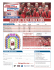### Bending Brakes FAQ - Van Mark Products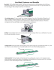### Oorsprong, verleden en toekomst van onze huisdieren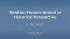### Information material for Van-Mark brakes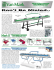### Trim-A-Brake II - Van Mark Products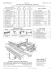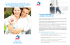### Neznámá pravda o společnosti Keshe Foundation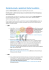### 1 - Marja van Putten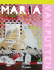### this publication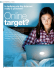### Desember 2015 Nuusbrief - Stellenbosch University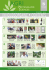### Apeldoorn 2007 Report - British Council | Netherlands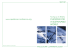### Introducing “Bead Form Dies” For TrimFormer™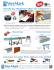### brochure - Habru Duivensport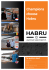### Appaloosa News Spot - appaloosa horse breeders` society of south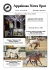### Mainfreight 2013 Annual Report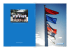### The Genus Dendrobium - St. Augustine Orchid Society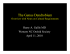### LIND Brochure 14102011